# Euros

Michal, Peter, John and Lenka got together 2,400 euros. They share an amount in ratio 2:6:4:3. How many got each of them?

Result

m =  320
p =  960
j =  640
l =  480

#### Solution:Leave us a comment of example and its solution (i.e. if it is still somewhat unclear...):Be the first to comment!## Next similar examples:

1. AgesJohn, Teresa, Daniel and Paul have summary 56 years. Their ages are in a ratio of 1:2:5:6. Determine how many years have each of them.
2. Composite ratioJakub, Aneta, and Lenka divided 1342 USD in the ratio 5/2: 3/10: 1/4. How much did Lenka take?
3. Three workersThree workers got paid for work. First earn € 50, the second € 100 and third € 60. For further work should receive together € 735. How do you divide that amount in order to be distributed in the same proportion as the first job?
4. DonutsFind how many donuts each student will receive if you share 126 donuts in a ratio of 1:5:8
5. PeppersIn the box are yellow (a), green (b) and red (c) peppers. Their amount is in a ratio 2:4:1 . Most are yellow peppers and green the least. Calculate the number of peppers each type if the total number of peppers is 70.
6. BicycleThe bicycle pedal gear has 36 teeth, the rear gear wheel has 10 teeth. How many times turns rear wheel, when pedal wheel turns 120x?
7. Salt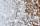1 t of sea water contains 25 kg of salt. How many tons of seawater must be evaporated to obtain 2 q of salt?
8. Lorry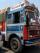The lorry was loaded with 18 boxes of 15 kg. How many boxes with weight 18 kg can be loaded, if total load must be same?
9. Ratio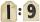Unfold number 1963 in the ratio 9:2:2.
10. Land areaA land area of Asia and Africa are in a 3: 2 ratio, the European and African are is 1:3. What are the proportions of Asia, Africa, and Europe?
11. Image scale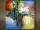The actual image dimensions are 60 cm x 80cm and has a reduced size 3 cm x 4 cm. At what scale the image was reduced?
12. Divide 5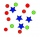Divide 288 in the following ratio 3 : 4 : 5
13. Line segmentThe 4 cm long line segment is enlarged in the ratio of 5/2. How many centimeters will measure the new line segment?
14. The squareThe square root of 25 times the square root of 81 is what number?
15. Double percent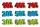What is 80% of 60% of 2800?
16. 1.5 divided1.5 divided by 1 = w divided by 4
17. Percent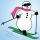From 99 children who participated in the ski course were 23 excellent skiers, 13 good and 20 average and the rest were beginners. Calculate this data in percentages.